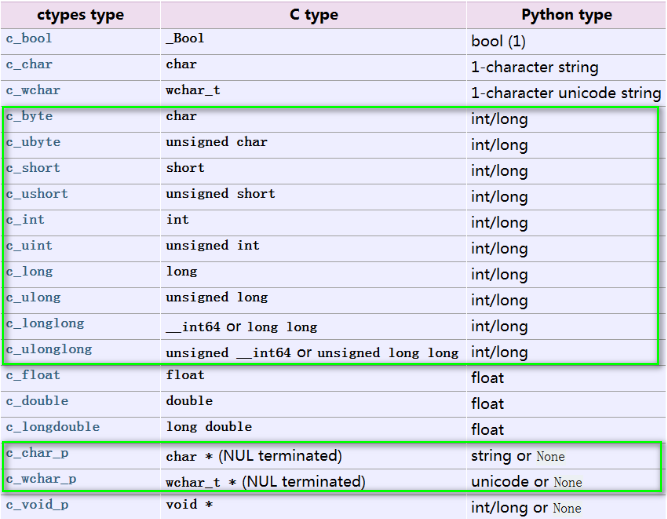# python3和c/c++的混合编程(ctypes的使用)

python作为一种胶水语言具有较高的开发效率, 但是其运行效率比较低, 当计算量比较大时,显得力不从心.  而ctypes是python下的一个可以链接c/c++的一个库. 可以将C函数编译成动态链接库, 即window下的.dll文件或者是linux下的.so文件. 这样我们通过使用cytpes可以加速代码的运行速度.

import platform
from ctypes import *

if platform.system() == 'Windows':
libc = cdll.LoadLibrary('msvcrt.dll')
elif platform.system() =='Linux':
libc = cdll.LoadLibrary('libc.so.6')

libc.printf('Hello world!\n')

1.数据类型, ctypes作为联系python和c的接口, 其对应的数据类型如下:None：对应 C 中的 NULL

intlong： 对应 C 中的 int，具体实现时会根据机器字长自动适配。

Byte String：对应 C 中的一个字符串指针 char * ，指向一块内存区域。(字符串前面会加小b,  b"helloworld")

Unicode String ：对应 C 中一个宽字符串指针 wchar_t *，指向一块内存区域。(对应的就是字符串, "helloworld")

2指定函数的参数类型和返回类型

#include <cmath>
#include <iostream>
using namespace std;

extern "C" float Dist(float ax,float ay,float az,float bx,float by,float bz){
return(sqrt((ax-bx)*(ax-bx)+(ay-by)*(ay-by)+(az-bz)*(az-bz)));}

test = cdll.LoadLibrary("./dist.so")
test.dist.argtypes = [c_float, c_float, c_float, c_float, c_float, c_float]
test.dist.restype = c_float
a =[3,0,0]
a1 = [0,4,0]
r = test.Dist(c_float(a),c_float(a),c_float(a), c_float(a1),c_float(a1),c_float(a1))

其中test.dist.argtypes = [c_float, c_float, c_float, c_float, c_float, c_float]表示函数的6个参数均为float型,
test.dist.restype = c_float函数的返回类型为float型.

3.结构体和联合体(Structures and unions)

>>> from ctypes import *
>>> class POINT(Structure):
...     _fields_ = [("x", c_int),
...                 ("y", c_int)]
...
>>> point = POINT(10, 20)
>>> print point.x, point.y
10 20
>>> point = POINT(y=5)
>>> print point.x, point.y
0 5
>>> POINT(1, 2, 3)
Traceback (most recent call last):
File "<stdin>", line 1, in <module>
ValueError: too many initializers
>>>